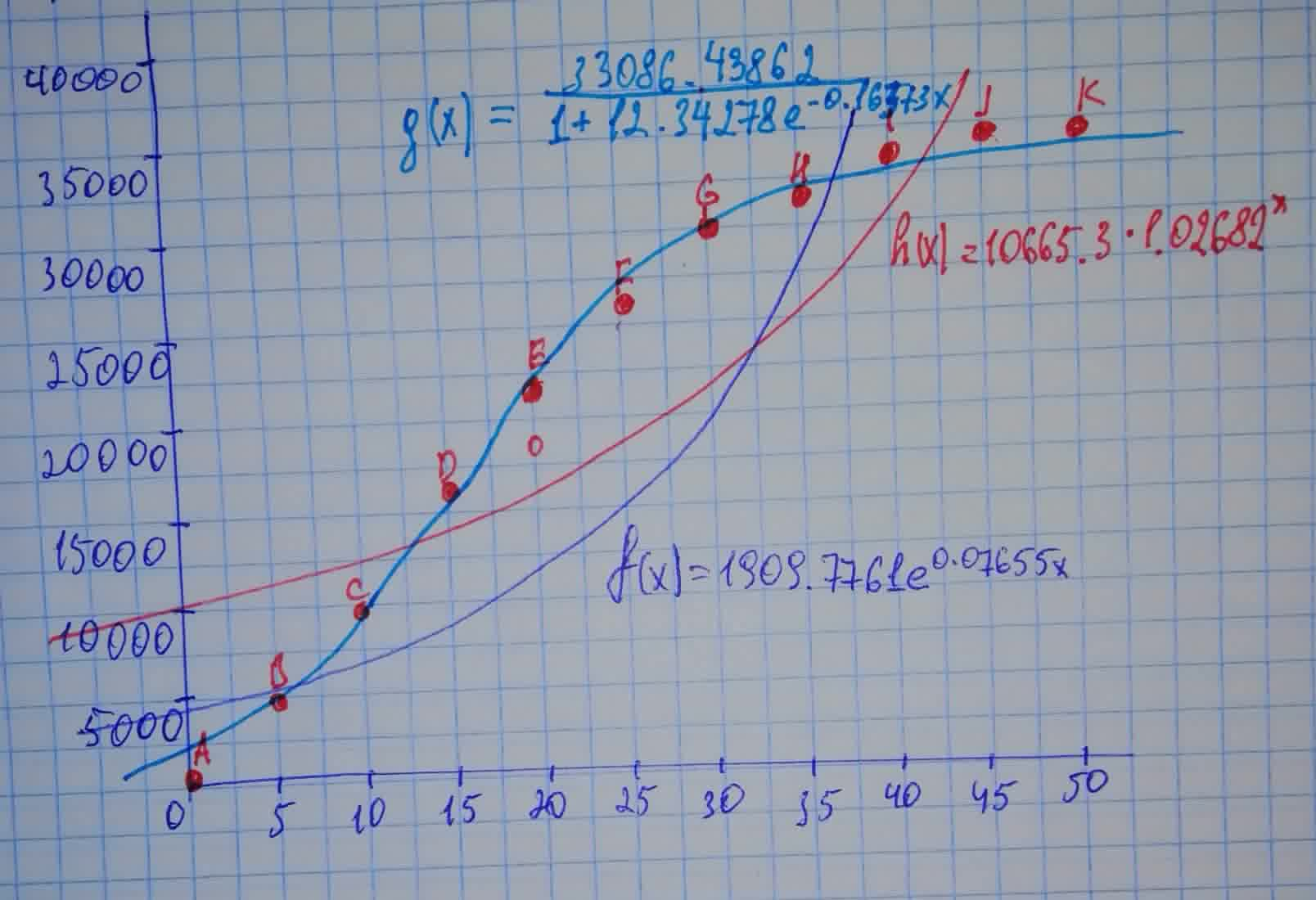# The table gives the midyear population of Japan, in thousands, from 1960 to 2010.Albarellak 2021-01-31 Answered

The table gives the midyear population of Japan, in thousands, from 1960 to 2010.
$\begin{array}{|cc|}\hline \text{Year}& \text{Population}\\ 1960& 94.092\\ 1965& 98.883\\ 1970& 104.345\\ 1975& 111.573\\ 1980& 116.807\\ 1985& 120.754\\ 1990& 123.537\\ 1995& 125.327\\ 2000& 126.776\\ 2005& 127.715\\ 2010& 127.579\\ \hline\end{array}$
Use a calculator to fit both an exponential function and a logistic function to these data. Graph the data points and both functions, and comment on the accuracy of the models. [Hint: Subtract 94,000 from each of the population figures. Then, after obtaining a model from your calculator, add 94,000 to get your final model. It might be helpful to choose $t=0$ to correspond to 1960 or 1980.]

You can still ask an expert for help

• Questions are typically answered in as fast as 30 minutes

Solve your problem for the price of one coffee

• Math expert for every subject
• Pay only if we can solve itcheekabooy

Step 1
Following the suggestions given by the problem, let
$t=$ the number of years after 1960 (so $t=0$ is 1960)
enter the population numbers with 94000 subtracted from each.
Using Desmos, first add a table. (clic on "$+$" at the upper left) and enter the numbers. It should look something like in this image:
Step 2
$\begin{array}{|cc|}\hline {x}_{1}& {y}_{1}\\ 0& 94092-94000\\ 5& 98883-94000\\ 10& 104345-94000\\ 15& 111573-94000\\ 20& 116807-94000\\ 25& 120754-94000\\ 30& 123537-94000\\ 35& 125327-94000\\ 40& 126776-94000\\ 45& 127715-94000\\ 50& 127579-94000\\ \hline\end{array}$

Step 3
Next, you can get an exponential form $y=a{b}^{x}$ by typing in the next box below the one with the table:

The values for a and b appear below. It should look similar to what is the lower right in the image above. Desmos gives a different answer than the book's. (Compare it in the graph at the bottom). The model with the proper variable names and shifted back up by 94000 would be:

Step 4
Next in another box below, type this to get a logistic model:

$k=0.165732$
Step 5
This time it does given an answer similar to the book's. Using the right variables and shifting back up by 94000, we have:

Step 6
Using GeoGebra 5 and entering the same numbers in as a list of points, the command FitExp does give the same answer as the book. (shifted it back up by 94000 here)

$=1909.7761\left({e}^{{0.07655}^{t}}\right)+94000$

Step 7
The logistic model is better than the exponential. The Desmos one seems to stay closer to the points overall than the other exponential one.